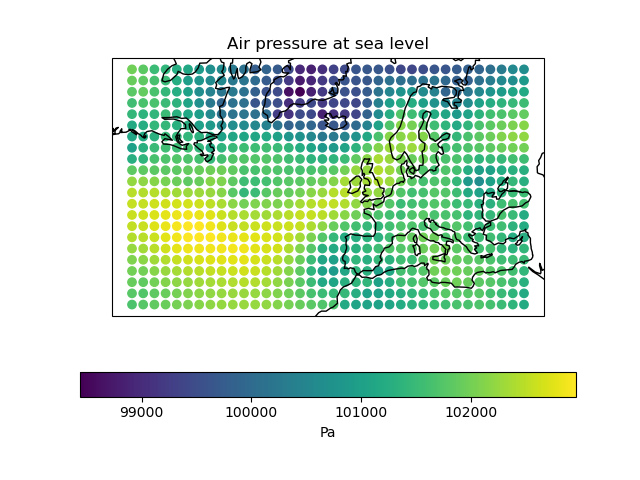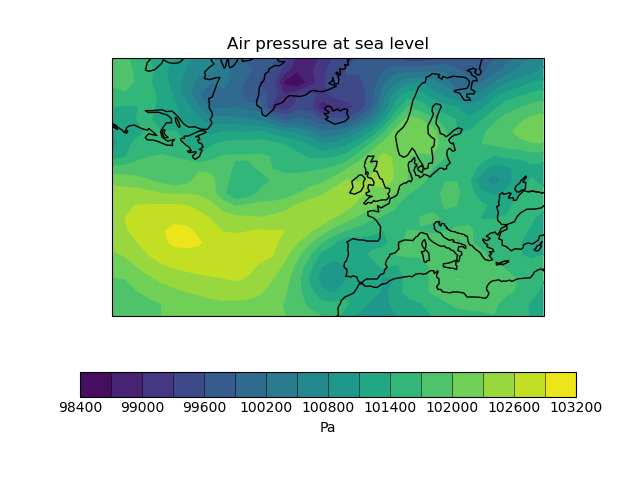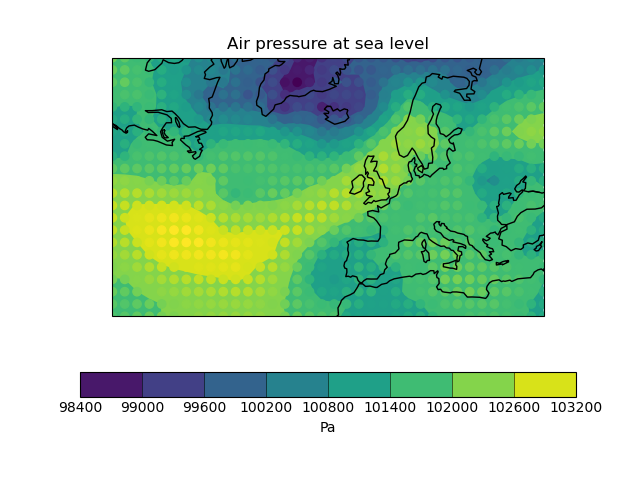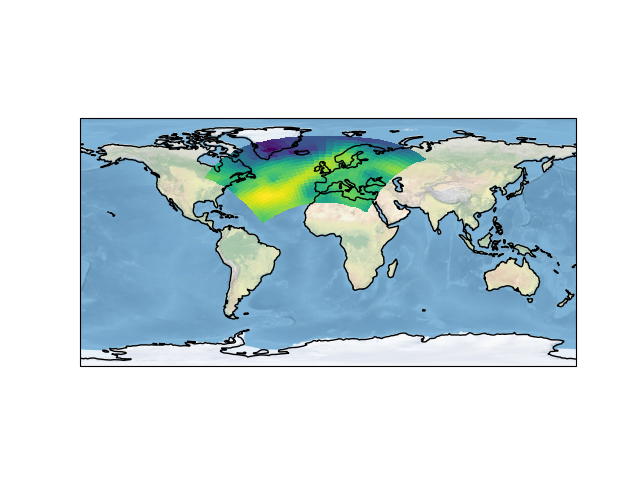# Rotated Pole Mapping#

This example uses several visualisation methods to achieve an array of differing images, including:

• Visualisation of point based data

• Contouring of point based data

• Block plot of contiguous bounded data

• Non native projection and a Natural Earth shaded relief image underlay

••••```import cartopy.crs as ccrs
import matplotlib.pyplot as plt

import iris
import iris.analysis.cartography
import iris.plot as iplt
import iris.quickplot as qplt

def main():
fname = iris.sample_data_path("rotated_pole.nc")

# Plot #1: Point plot showing data values & a colorbar
plt.figure()
points = qplt.points(air_pressure, c=air_pressure.data)
cb = plt.colorbar(points, orientation="horizontal")
cb.set_label(air_pressure.units)
plt.gca().coastlines()
iplt.show()

# Plot #2: Contourf of the point based data
plt.figure()
qplt.contourf(air_pressure, 15)
plt.gca().coastlines()
iplt.show()

# Plot #3: Contourf overlaid by coloured point data
plt.figure()
qplt.contourf(air_pressure)
iplt.points(air_pressure, c=air_pressure.data)
plt.gca().coastlines()
iplt.show()

# For the purposes of this example, add some bounds to the latitude
# and longitude
air_pressure.coord("grid_latitude").guess_bounds()
air_pressure.coord("grid_longitude").guess_bounds()

# Plot #4: Block plot
plt.figure()
plt.axes(projection=ccrs.PlateCarree())
iplt.pcolormesh(air_pressure)
plt.gca().stock_img()
plt.gca().coastlines()
iplt.show()

if __name__ == "__main__":
main()
```

Total running time of the script: ( 0 minutes 0.812 seconds)

Gallery generated by Sphinx-Gallery